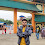# Hackerrank Minimum swaps 2 problem solution

In this HackerRank Minimum swaps 2 problem, we need to develop a program that accepts an array consisting of integers without any duplicates.  and we only allowed to swap any two elements. we need to print out the minimum number of swaps required to sort an array in ascending order.

## Problem solution in Python 2 programming.

```#!/bin/python

import math
import os
import random
import re
import sys

# Complete the minimumSwaps function below.
def minimumSwaps(arr):
temp =  * (len(arr) + 1)
for pos, val in enumerate(arr):
temp[val] = pos
pos += 1
swaps = 0
for i in range(len(arr)):
if arr[i] != i+1:
swaps += 1
t = arr[i]
arr[i] = i+1
arr[temp[i+1]] = t
temp[t] = temp[i+1]
return swaps

if __name__ == '__main__':
fptr = open(os.environ['OUTPUT_PATH'], 'w')

n = int(raw_input())

arr = map(int, raw_input().rstrip().split())

res = minimumSwaps(arr)

fptr.write(str(res) + '\n')

fptr.close()

```

## Problem solution in Python 3 programming.

```#!/bin/python3

import math
import os
import random
import re
import sys

# Complete the minimumSwaps function below.
def minimumSwaps(arr):
temp =  * (len(arr) + 1)
for pos, val in enumerate(arr):
temp[val] = pos
pos += 1
swaps = 0
for i in range(len(arr)):
if arr[i] != i+1:
swaps += 1
t = arr[i]
arr[i] = i+1
arr[temp[i+1]] = t
temp[t] = temp[i+1]
return swaps

if __name__ == '__main__':
fptr = open(os.environ['OUTPUT_PATH'], 'w')

n = int(input())

arr = list(map(int, input().rstrip().split()))

res = minimumSwaps(arr)

fptr.write(str(res) + '\n')

fptr.close()

```

### Problem solution in java programming.

```import java.io.*;
import java.math.*;
import java.security.*;
import java.text.*;
import java.util.*;
import java.util.concurrent.*;
import java.util.regex.*;

public class Solution {

// Complete the minimumSwaps function below.
static int minimumSwaps(int[] arr) {
int i =0;
int count=0;
int temp;
int  n = arr.length;
while(i<n){
if(arr[i] != i+1){
temp = arr[i];
arr[i] = arr[temp-1];
arr[temp-1]=temp;
count++;
}
else{
i++;
}
}
return count;
}

private static final Scanner scanner = new Scanner(System.in);

public static void main(String[] args) throws IOException {
BufferedWriter bufferedWriter = new BufferedWriter(
new FileWriter(System.getenv("OUTPUT_PATH")));

int n = scanner.nextInt();
scanner.skip("(\r\n|[\n\r\u2028\u2029\u0085])?");

int[] arr = new int[n];

String[] arrItems = scanner.nextLine().split(" ");
scanner.skip("(\r\n|[\n\r\u2028\u2029\u0085])?");

for (int i = 0; i < n; i++) {
int arrItem = Integer.parseInt(arrItems[i]);
arr[i] = arrItem;
}

int res = minimumSwaps(arr);

bufferedWriter.write(String.valueOf(res));
bufferedWriter.newLine();

bufferedWriter.close();

scanner.close();
}
}```

Note: In the above code while creating the Bufferwriter object I shifted the code into another line.

1.I have been reading this code over and over again, trying to break it down but am super confused right after you create a list of 0s the length of the array. Is there any chance you could annotate what is going on here?

1.can you please tell me which solution you are reading in which programming.

2.1.